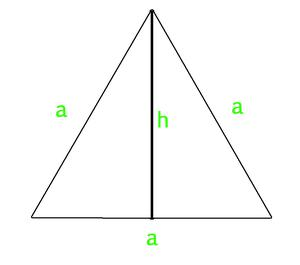GFG App
Open AppBrowser
Continue

# Program to calculate area and perimeter of equilateral triangle

An equilateral triangle is a triangle in which all three sides and angles are equal. All three internal angles of equilateral triangle measures 60 degree.

• If we know the length of each sides of equilateral triangle, then we can use below mentioned formula to calculate area of equilateral triangle.
`Area of Equilateral Triangle = (sqrt(3)/4) * a * a    `
• If we know the length of altitude of equilateral triangle along with the length of side, then we can use below mentioned formula to calculate it’s area.
`Area of Equilateral Triangle = (1/2) x Side x Altitude`

Perimeter of Equilateral Triangle :

`Perimeter of Equilateral Triangle :  3 X a`

How does the area formula work?
Let us take a look at below diagram. We know are of a triangle is 1/2 * base * height. The value of h is sqrt(a2 – (a/2)2) = sqrt(3) * a / 2. So the area becomes 1/2 * a * (sqrt(3) * a / 2) = (sqrt(3)/4) * a * aExamples:

```Input : side = 4
Output : Area of Equilateral Triangle: 6.9282
Perimeter of Equilateral Triangle: 12
Input : side = 12
Output : Area of Equilateral Triangle: 62.3538
Perimeter of Equilateral Triangle: 36```

## C++

 `// CPP program to find area ` `// and perimeter of equilateral triangle ` `#include ` `using` `namespace` `std; ` ` `  `// Function to calculate Area ` `// of equilateral triangle ` `float` `area_equi_triangle(``float` `side) ` `{ ` `    ``return` `sqrt``(3) / 4 * side * side; ` `} ` ` `  `// Function to calculate Perimeter ` `// of equilateral triangle ` `float` `peri_equi_triangle(``float` `side) ` `{ ` `    ``return` `3 * side; ` `} ` ` `  `// Driver Code ` `int` `main() ` `{ ` `    ``float` `side = 4; ` `    ``cout << ``"Area of Equilateral Triangle: "` `        ``<< area_equi_triangle(side) << endl; ` `    ``cout << ``"Perimeter of Equilateral Triangle: "` `        ``<< peri_equi_triangle(side); ` `    ``return` `0; ` `} `

## Java

 `// Java Program to find area and ` `// perimeter of equilateral triangle ` `import` `java.io.*; ` ` `  `class` `GFG ` `{ ` `    ``// Function to calculate ` `    ``// Area of equilateral triangle ` `    ``static` `float` `area_equi_triangle(``float` `side) ` `    ``{ ` ` `  `        ``return` `(``float``)(((Math.sqrt(``3``)) / ``4``) * ` `                        ``side * side); ` `    ``} ` ` `  `    ``// Function to calculate ` `    ``// Perimeter of equilateral ` `    ``// triangle ` `    ``static` `float` `peri_equi_triangle(``float` `side) ` `    ``{ ` `        ``return` `3` `* side; ` `    ``} ` `     `  `    ``// Driver Code ` `    ``public` `static` `void` `main(String arg[]) ` `    ``{ ` `        ``float` `side = ``4``; ` `        ``System.out.print(``"Area of Equilateral Triangle:"``); ` `        ``System.out.println(area_equi_triangle(side)); ` `        ``System.out.print(``"Perimeter of Equilateral Triangle:"``); ` `        ``System.out.println(peri_equi_triangle(side)); ` `    ``} ` `} ` ` `  `// This code is contributed ` `// by Anant Agarwal. `

## Python

 `# Python3 program to calculate Area and ` `# Perimeter of equilateral Triangle ` ` `  `# Importing Math library for sqrt ` `from` `math ``import` `*` ` `  `# Function to calculate Area ` `# of equilateral triangle ` `def` `area_equilateral( side ): ` `    ``area ``=` `(sqrt(``3``) ``/` `4``) ``*` `side ``*` `side ` `    ``print` `(``"Area of Equilateral Triangle: % f"``%` `area) ` ` `  `# Function to calculate Perimeter ` `# of equilateral triangle ` `def` `perimeter( side ): ` `    ``perimeter ``=` `3` `*` `side ` `    ``print` `(``"Perimeter of Equilateral Triangle: % f"``%` `perimeter) ` `     `  `# Driver code ` `side ``=` `4` `area_equilateral( side ) ` `perimeter( side ) `

## C#

 `// C# Program to find area and ` `// perimeter of equilateral triangle ` `using` `System; ` ` `  `class` `GFG ` `{ ` `    ``// Function to calculate ` `    ``// Area of equilateral triangle ` `    ``static` `float` `area_equi_triangle(``float` `side) ` `    ``{ ` ` `  `        ``return` `(``float``)(((Math.Sqrt(3)) / 4) * ` `                        ``side * side); ` `    ``} ` ` `  `    ``// Function to calculate ` `    ``// Perimeter of equilateral ` `    ``// triangle ` `    ``static` `float` `peri_equi_triangle(``float` `side) ` `    ``{ ` `        ``return` `3 * side; ` `    ``} ` `     `  `    ``// Driver Code ` `    ``public` `static` `void` `Main() ` `    ``{ ` `        ``float` `side = 4; ` `        ``Console.Write(``"Area of Equilateral Triangle:"``); ` `        ``Console.WriteLine(area_equi_triangle(side)); ` `        ``Console.Write(``"Perimeter of Equilateral Triangle:"``); ` `        ``Console.WriteLine(peri_equi_triangle(side)); ` `    ``} ` `} ` ` `  `// This code is contributed ` `// by vt_m. `

## PHP

 ` `

## Javascript

 ``

Output

```Area of Equilateral Triangle: 6.9282
Perimeter of Equilateral Triangle: 12```

Time Complexity: O(1)

Auxiliary Space: O(1)

My Personal Notes arrow_drop_up